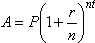# The Modern Survival Guide #70

## Exponents: What They Do to Investments and DebtsWhere A is the ending amount, P is the principal, r is the interest rate in decimals, n is the number of compoundings per year, and t is the number of years

Written by